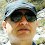## Friday, September 2, 2011

### How to make up any value of resistor for replacement if you can’t get the exact value

On your line of duty as a technician in electronics sometimes you may come across a resistor burnt or has changed its original value and being one of the cheapest component in the market you visit your nearest electronics shop but alas, they can’t get the exact resistor value you are looking for.

I have in mind the example of the current sense resistor which is found in almost all switch mode power supply. Current sense resistors are usually low ohm and very precise and therefore a small change in its original value (usually upwards) can cause the whole power supply to appear dead.

In this lesson I am going to talk a bit on how you can make up any value of resistor you need for replacement in case you can’t get the exact value. In order to accomplish this mission we are going to use some characteristic of resistors when connected in series and in parallel.

If resistors are connected in series then their individual resistance are added together to get the total resistance. See picture below.

If resistors are connected in parallel their total resistance is always less than the smallest resistor value in the association. In the example below we have two one(1 Ohm) resistors in parallel. If the resistance value is the same in parallel association then the formula is simple. Just divide the value of one resistor by the number of resistors.

From the above picture we have the value of the resistor is One(1 Ohm), to get the total value of resistance in parallel just divide the 1 ohm by the number of resistors two(2) and the value is ½ or 0.5 ohms.

As you can see the total resistance value is less than the smallest value resistor (in our case one (1 ohm). Therefore if you wanted to get half ohm resistor there you are.

So next time you encounter problem of getting a certain resistor value now you know how to go round this problem.

All the best Guys

See you in the next class

1.HI HUMPHREY

yes .in power supply of mentioned PARS GRUNDIG TV
that i repaired and i post the report to you is also one 1/2 watt 1.2 OHM resistor is employeed just for detection of current and sensing how much the circute drive current and most time this resistor blow just like fusiable resistor and indicat some thing is worng in the circuit. ofcourse deeper analize of this important resistor is requierd
regards
beh

2.sure Beh, but i haven't seen this resistor rated 1/2 watt. its always 2 watts so it usually big in size.
regards Humphrey

3.HI HUMPHREY
ok i will try to send you a picture of this resistor. of course on the board
beh

4.Humphrey

5.If you may be looking for legacy parts, you might want to try www.stereorepair.com

6.pls how do i know accurately the value of a burnt resistor plssssssssssssssssssssssssssssssss?

1.